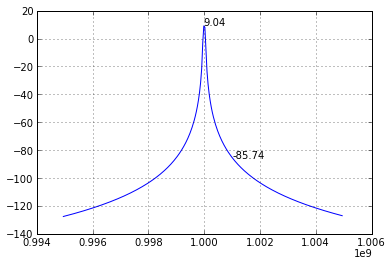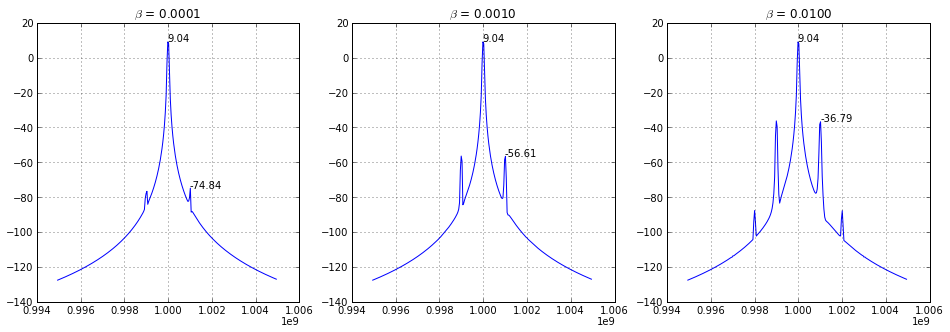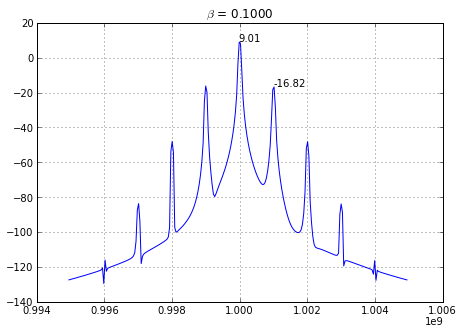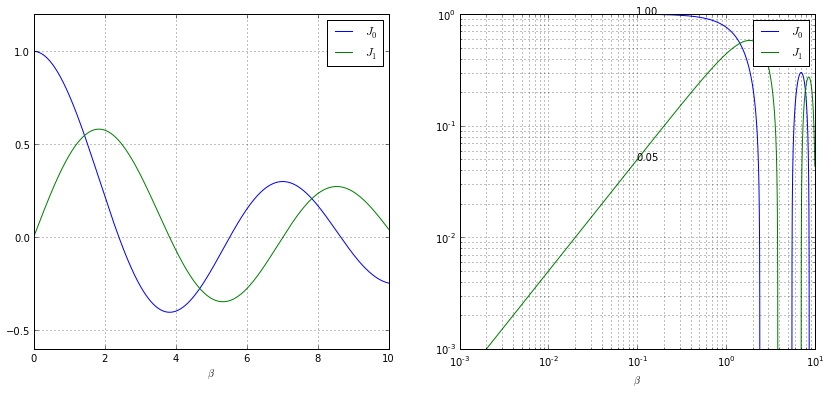Overview:
A Real-Time sampling oscilloscope is designed to capture time-domain phenomena but the combination of an extremely accurate timebase and Fast Fourier Transforms can make them very useful at measuring frequency domain phenomena.
Phase Noise is traditionally measured using a frequency domain instrument such as a Spectrum Analyzer or specialised Signal Source Analyzer. While these frequency domain instruments typically have high dynamic range there are limitations to their measurement capability in terms of the offset frequency they are capable of measuring to or the fact they are unable to measure the phase noise of a non-clock signal.

This note will explain how to measure the phase noise of a clock or data signal using an Agilent Infiniium real-time sampling oscilloscope using a tool called Infiniium Phase Noise.

First a bit of background on Phase Modulation PM.
Although phase noise refers to the uncorrelated portion of the phase modulation it is informative to take the simple example of sinusoidal phase modulation to build our intuition first.

$$y(t) = sin(\omega_c t + \phi(t))$$

• $\omega_c = 2pif_c$
• $f_c$ is the frequency of the carrier sinusoid

• $$\phi(t) = \beta cos(\omega_m t)$$
• $\beta$ is the peak phase deviation [rads] of the phase modulation
• $\omega_m = 2pif_m$
• $f_m$ is the frequency of the modulating signal
</ul> </ul>
We can simulate the spectra of some simple signals and then confirm with some measurements.

In :
f_c = 1e9 # The carrier frequency 1GHz
sa_rate = 80e9 # Sample rate 80GSa/s
mem_depth = 2**21 # Number of samples to take ~ 2 million (a power of 2 makes fft's simpler)
t = linspace(0,mem_depth/sa_rate,mem_depth) # Create the time sample array

def plot_wfm_spectrum(t,dt,f_c,beta,f_m):
"""Since we'll create a few plots of similar waveforms let's not duplicate our work"""
v = sin(2*pi*f_c*t + beta*cos(2*pi*f_m*t))
window = hanning(mem_depth)
v_windowed=v*window # Apply a Hanning window to improve the spectrum
v_spec = fft.fft(v_windowed)
freqs = fft.fftfreq(mem_depth, 1/sa_rate)
dfreqs = freqs
f_freq_idx = round(f/dfreqs)
span = 10*f_mod
f_min_idx = int(f_freq_idx - span/(2*dfreqs))
f_max_idx = int(f_freq_idx + span/(2*dfreqs))
psd_v_spec = 20*log10(2*sqrt(2)*abs(v_spec)/(len(v)*0.2236))
plot(freqs[f_min_idx:f_max_idx],psd_v_spec[f_min_idx:f_max_idx])
f_c_idx = round(f_c/dfreqs)
annotate("{:.2f}".format(psd_v_spec[f_c_idx]),(freqs[f_c_idx],psd_v_spec[f_c_idx]))
f_m_idx = round((f_c+f_m)/dfreqs)
annotate("{:.2f}".format(psd_v_spec[f_m_idx]),(freqs[f_m_idx],psd_v_spec[f_m_idx]))
grid(1)

# First let's look at the spectrum of the carrier with no modulation
beta = 0 # Phase Modulation, PM
f_m = 1e6
plot_wfm_spectrum(t,dt,f_c,beta,f_m)
grid(1)In :
# Let's add some sinusoidal Phase Modulation @ 1MHz
f_m = 1e6
figure(figsize = (16,5))
subplot(1,3,1)
beta = 0.0001
title(r"$\beta$ = %.4f" %beta)
plot_wfm_spectrum(t,dt,f_c,beta,f_m)
subplot(1,3,2)
beta = 0.001
title(r"$\beta$ = %.4f" %beta)
plot_wfm_spectrum(t,dt,f_c,beta,f_m)
subplot(1,3,3)
beta = 0.01
title(r"$\beta$ = %.4f" %beta)
plot_wfm_spectrum(t,dt,f_c,beta,f_m)Note that at first the modulation sideband at +/- 1MHz increases linearly (+20dB for a 10x step) with increase in the modulation amplitude. Eventually other sidebands appear at +/- N MHz.

In :
# Let's compare a measurement at large modulation amplitude with a measurement from a scope
f_m = 1e6
beta = 0.1
figure(figsize = (16,5))
subplot(1,2,1)
title(r"$\beta$ = %.4f" %beta)
plot_wfm_spectrum(t,dt,f_c,beta,f_m)
#from visa import *
#scope = instrument("TCPIP0::130.30.240.189::inst0::INSTR")
#scope.write(":wav:sour func2")
#wfm = wfm_ascii[:-1].split(',')
#wfm_freqs = linspace(wfm_xor, len(wfm)*wfm_dx, len(wfm))
#subplot(1,2,2)
#title("PSD from Scope FFT - PM Amplitude = %.4f" %pm_amp)
#plot(wfm_freqs, wfm)
#grid(1)Note that the first modulation sideband is down ~26dB from the carrier.

The amplitudes of these sidebands can be predicted using Bessel Functions.

$$y(t) = sin(\omega_c t + \beta cos(\omega_\phi t))$$

It can be shown that our signal:

$$sin(\omega_c t + \beta cos(\omega_\phi t))$$

$$= J_0 (\beta) cos(\omega_c t)$$ $$- J_1 (\beta) (cos(\omega_c - \omega_m)t - cos(\omega_c + \omega_m)t)$$ $$+ J_2 (\beta) (cos(\omega_c - 2\omega_m)t - cos(\omega_c + 2\omega_m)t)$$ $$- ...$$
Where $J_0$, $J_1$, $J_2$ are Bessel Functions.

$J_0$ sets the amplitude of the carrier $f_c$
$J_1$ sets the amplitude of the 1st sidebands at $\pm f_m$
$J_2$ sets the amplitude of the 2nd sidebands at $\pm 2f_m$

So what do the Bessel functions look like?

In :
beta_marker = 0.1
from scipy.special import j0,j1
beta=linspace(0,10,10000)
dbeta = beta
y0=j0(beta)
y1=j1(beta)
figure(figsize=(14,6))
subplot(1,2,1)
plot(beta,y0,label="$J_0$")
plot(beta,y1,label="$J_1$")
legend()
xlabel(r"$\beta$")
grid(1)
subplot(1,2,2)
loglog(beta,y0,label="$J_0$")
loglog(beta,y1,label="$J_1$")
beta_marker_idx = beta_marker/dbeta
annotate("{:.2f}".format(y0[beta_marker_idx]),(beta_marker,y0[beta_marker_idx]))
annotate("{:.2f}".format(y1[beta_marker_idx]),(beta_marker,y1[beta_marker_idx]))
print y1[beta_marker]
legend()
ylim(ymin=1e-3)
xlabel(r"$\beta$")
grid(which='both')

0.0What does this tell us?
$J_0$ tells us the carrier amplitude and $J_1$ tells us the first sideband amplitude
If $\beta = 0$ (no modulation) $J_1$ (first sideband) is at zero amplitude relative to the carrier.
If $\beta \approx 2$ (peak phase deviation ~ 2 rads) the first sideband is actually larger than the carrier.

In our example where $\beta = 0.1$ rad, the carrier peak was at +9.01dBm & the first sideband at -16.8dBm for a delta of -26dB
This matches well with the Bessel function values of:
$J_0 = 1.0$, $J_1 = 0.05$

$20log10(0.05/1.0) = -26 dB$

What we are looking at in both of these plots is the power spectral density PSD of the signal, $S(f)$.
When a Spectrum Analyzer makes a Phase Noise measurement it starts with this $S(f)$ data. If there is no Amplitude Modulation of the signal we can call say $S(f) = S_\phi(f)$, the Frequency Spectral Density.

To get to from $S_\phi(f)$ to $L(f_\phi)$ we need to:

1. Normalize to a 1Hz resolution bandwidth
2. Normalize to the total power of the signal
3. Plot the data versus offset frequency from the carrier

This is the NIST definition of Phase Noise.

Another approach is to first de-modulate the phase from the signal and then calculate the Power Spectral Density of the phase, $S_\phi(f_\phi)$
An advantage of this approach is that it is more immune to amplitude modulation on the signal. The Signal Source Analyzer and dedicated Phase Noise test systems use this approach with a hardware phase demodulater. However a real-time oscilloscope can also demodulate phase using a software clock recovery algorithm to find the phase deviation, ph(t). If we then take the Fourier Transform of this we get the PSD of the phase modulation, $S_\phi(f_\phi)$

Translation of Bessel Functions

$$y(t) = sin(\omega_c t + \beta cos(\omega_\phi t))$$

From: $$sin(A + B) = sin(A)cos(B) + cos(a)sin(B)$$

$A = \omega_c t$; $B = \beta cos(\omega_\phi t)$

$y(t) = sin(\omega_c t)cos(\beta cos(\omega_\phi t)) + cos(\omega_c t)sin(\beta cos(\omega_\phi t))$

From: $sin(A)cos(B) = 1/2(sin(A-B)+sin(A+B))$

$y(t) = 1/2(sin(\omega_c t - \beta cos(\omega_\phi t)) + sin(\omega_c t + \beta cos(\omega_\phi t))) + 1/2(sin(\beta cos(\omega_\phi t)-\omega_c t) + sin(\beta cos(\omega_\phi t)+\omega_c t))$

In [ ]: